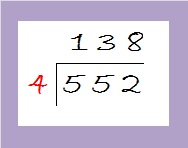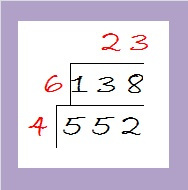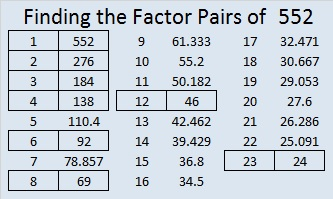# Level 2 and Simplifying √552

552 is the sum of all the prime numbers from 37 to 73.

It is also the sum of all the prime numbers from 79 to 103.

That means if you add up all the prime numbers from 37 to 103, you’ll get 1104 which is 2 x 552.

Can you list all those prime numbers?Print the puzzles or type the solution on this excel file: 12 Factors 2015-07-13

—————————————————————————————————

I’ve modified the cake method to help me find √552.  My philosophy is that there is no reason to completely break up 552 to find its square root when we’re just going to have to put it mostly back together again to give the final answer. About 83% of numbers whose square roots can be reduced are divisible by 4 and/or 9. It makes sense to check to see if 552 is divisible by either of those numbers first.

552 can be evenly divided by 4 because its last two digits are divisible by 4. That means √552 can be reduced. Let’s find 552 ÷ 4.The quotient is 138 which is even but not divisible by 4. Let’s check to see if it is divisible by nine: 1 + 3 + 8 = 12. It is divisible by 3, but not by 9.

Since 138 is even and divisible by 3, it is divisible by 6. It is easier to divide by 6 once than it is to divide first by 2 and then by 3. Dividing by 6 makes our division cake look like this:Since 23 is a prime number, we are done with the division process. Taking the square root of everything on the outside of the cake we get √552 = (√4)(√6)(√23) = (√4)(√138) = 2√138.

—————————————————————————————————

• 552 is a composite number.
• Prime factorization: 552 = 2 x 2 x 2 x 3 x 23, which can be written 552 = (2^3) x 3 x 23
• The exponents in the prime factorization are 3, 1, and 1. Adding one to each and multiplying we get (3 + 1)(1 + 1)(1 + 1) = 4 x 2 x 2 = 16. Therefore 552 has exactly 16 factors.
• Factors of 552: 1, 2, 3, 4, 6, 8, 12, 23, 24, 46, 69, 92, 138, 184, 276, 552
• Factor pairs: 552 = 1 x 552, 2 x 276, 3 x 184, 4 x 138, 6 x 92, 8 x 69, 12 x 46, or 23 x 24
• Taking the factor pair with the largest square number factor, we get √552 = (√4)(√138) = 2√138 ≈ 23.49468—————————————————————————————————1.Joseph Nebus
2.ivasallay